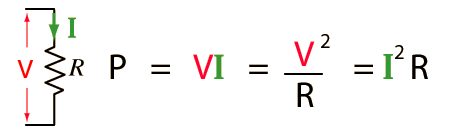# DC Electric Power

The electric power in watts associated with a complete electric circuit or a circuit component represents the rate at which energy is converted from the electrical energy of the moving charges to some other form, e.g., heat, mechanical energy, or energy stored in electric fields or magnetic fields. For a resistor in a DC Circuit the power is given by the product of applied voltage and the electric current:

P = VI
Power = Voltage x Current

watts = volts x amperes

Enter data in any two of the boxes, then click on the active text for the quantity you wish to calculate.

The details of the units are as follows:Power in a resistor AC Power
 Example of power in series and parallel circuits
Index

DC Circuits

 HyperPhysics***** Electricity and Magnetism R Nave
Go Back

# Power Dissipated in Resistor

Convenient expressions for the power dissipated in a resistor can be obtained by the use of Ohm's Law.These relationships are valid for AC applications also if the voltages and currents are rms or effective values. The resistor is a special case, and the AC power expression for the general case includes another term called the power factor which accounts for phase differences between the voltage and current.

The fact that the power dissipated in a given resistance depends upon the square of the current dictates that for high power applications you should minimize the current. This is the rationale for transforming up to very high voltages for cross-country electric power distribution.

Index

DC Circuits

 HyperPhysics***** Electricity and Magnetism R Nave
Go Back

# DC Power in Series and Parallel Circuits

The power relationship is one of the main tools for the analysis of electric circuits, along with Ohm's Law, the voltage law and the current law. Applying the current law to the above circuits along with Ohm's law and the rules for combining resistors gives the numbers shown below. The determining of the voltages and currents associated with a particular circuit along with the power allows you to completely describe the electrical state of a direct current circuit.Index

DC Circuits

 HyperPhysics***** Electricity and Magnetism R Nave
Go Back